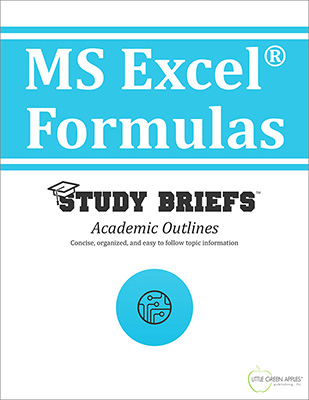# MS Excel FormulasLook inside
• Format: eBook
• Edition: 2016
• Publisher: Little Green Apples Publishing LLC

Price: \$4.99

## Description:

The MS Excel® Formulas Study Brief provides the end-user with a handy reference guide that describes useful and time-saving formulas for this widely-used spreadsheet application. This Study Brief collects and organizes a wealth of need-to-know information in a short format. It is intended to serve as a basic introduction for new users of Microsoft Excel® that want to be more productive quickly, or as a refresher for experienced Excel® users to findd additional tips and techniques for working more efficiently. A quick review of Excel® formula basics outlines some key fundamentals, including parts of an MS Excel® formula, types of formulas, functions and operators, how to enter formulas and how to correct common errors. Sample formulas are categorized by function in useful reference charts, providing proper syntax, explanations of use, and real-world examples with actual data. Helpful formulas for working with statistical and math functions, date and time functions, text functions, lookup and reference functions, information functions and logical functions are included. Details about complex and combination formulas are also provided for the advanced user.

## Topics Covered:

• What are MS Excel® Formulas?
• Parts of a MS Excel® Formula
• How to Enter Formulas
• Types of Formulas
• Cell References
• Types of Operators
• Errors in Formulas
• Working with Formulas
• Complex/Combination Formulas

Browse Subjects